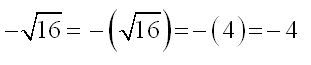## Friday, July 24, 2009

### Common Math Mistake 4

Proper Use of Square Root
There seems to be a very large misconception about the use of square roots out there.
Students seem to be under the misconception thatThis is not correct however. Square roots are ALWAYS positive! So the correct value isThis is the ONLY value of the square root! If we want the -4 then we do the followingNotice that I used parenthesis only to make the point on just how the minus sign was appearing! In general the middle two steps are omitted. So, if we want the negative value we have to actually put in the minus sign!

Here is the proper solution technique for this problem.Note that the ± shows up in the second step before we actually find the value of the square root! It doesn’t show up as part of taking the square root.

So remember that square roots ALWAYS return a positive answer. If you want a negative you’ll need to put it in a minus sign BEFORE you take the square root.

### NUMBER OF TRAILING ZEROES IN A PRODUCT (PART -2)

Dear all, Please do watch my latest video (dated 10-01-2021) on the topic “NUMBER OF TRAILING ZEROES IN A PRODUCT (PART -2)” VIDEO LINK HERE...